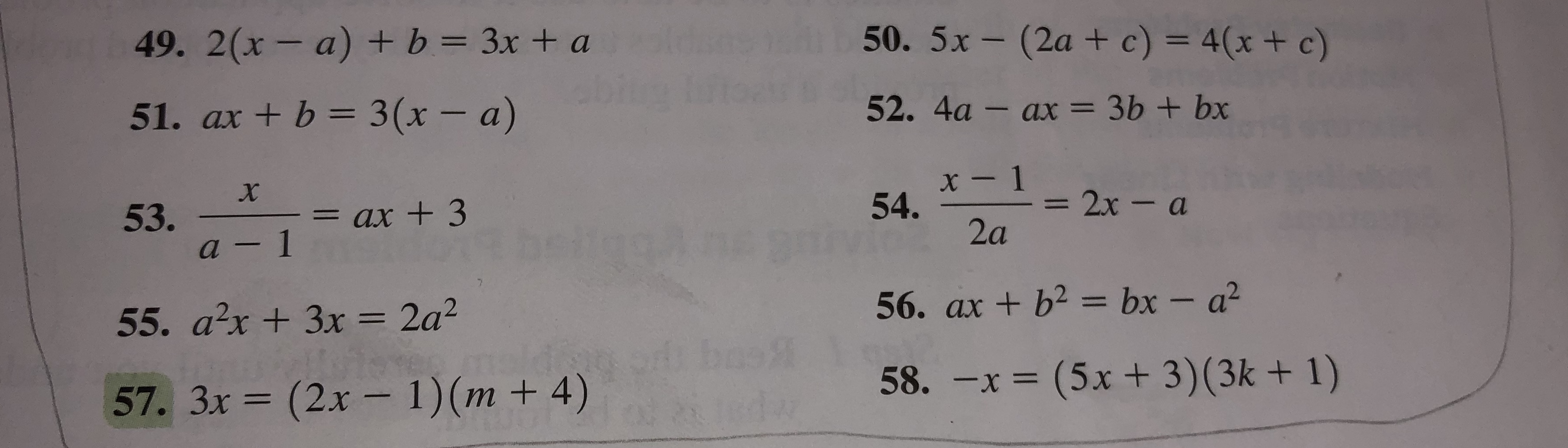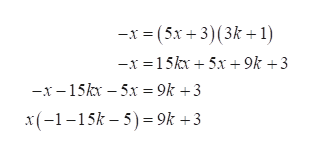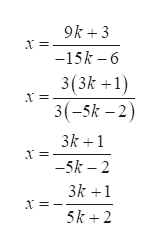# 50. 5x-(2a + c) = 4(x + c)49. 2(x- a) + b = 3x + a52. 4a - ax = 3b + bx51. ax + b 3 (x - a)x- 154.2x-a53.a-1= ax +32a56. ax +b2 bx - a255. a2x+3x = 2a258. -x = (5x+ 3)(3k + 1)(2x- 1)(m + 4)57. 3x(3

Question
9 views

I need assistance in solving question 58.help_outlineImage Transcriptionclose50. 5x-(2a + c) = 4(x + c) 49. 2(x- a) + b = 3x + a 52. 4a - ax = 3b + bx 51. ax + b 3 (x - a) x- 1 54. 2x-a 53. a-1 = ax +3 2a 56. ax +b2 bx - a2 55. a2x+3x = 2a2 58. -x = (5x+ 3)(3k + 1) (2x- 1)(m + 4) 57. 3x (3 fullscreen
check_circle

Step 1

58. The given equation is

Step 2

Simplify the equation as follows.help_outlineImage Transcriptionclose-x(5x+3) (3k1) -x =15k 5x + 9k +3 -x-15k -5x= 9k +3 x(--15k5 9k +3 fullscreen
Step 3

Simplify fur...help_outlineImage Transcriptionclose9k 3 -15k -6 3(3k +1) 3(-5k -2) 3k 1 -5k 2 3k 1 5k 2 fullscreen

### Want to see the full answer?

See Solution

#### Want to see this answer and more?

Solutions are written by subject experts who are available 24/7. Questions are typically answered within 1 hour.*

See Solution
*Response times may vary by subject and question.
Tagged in

### Other